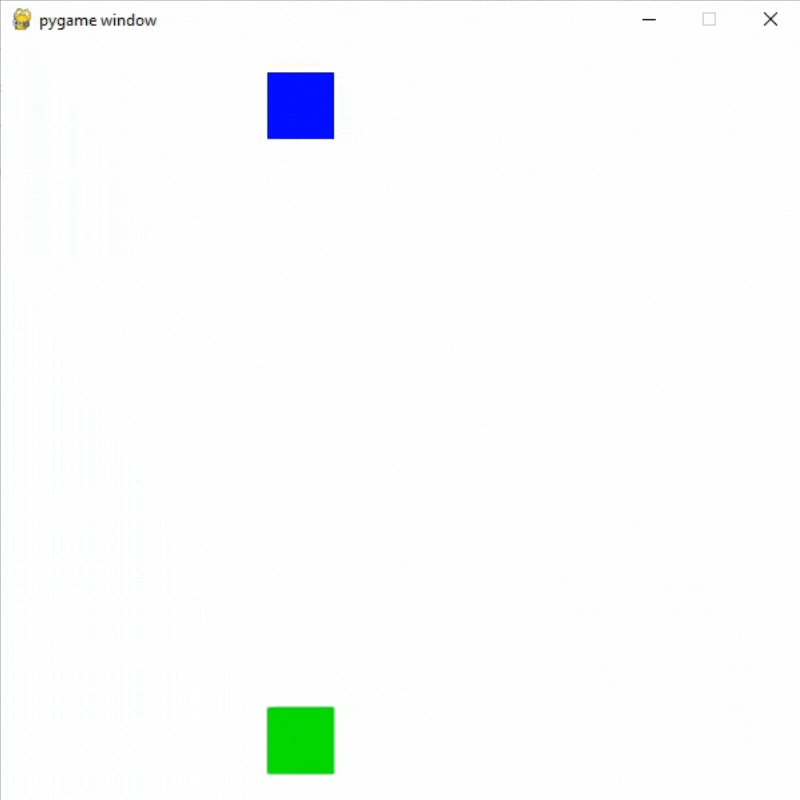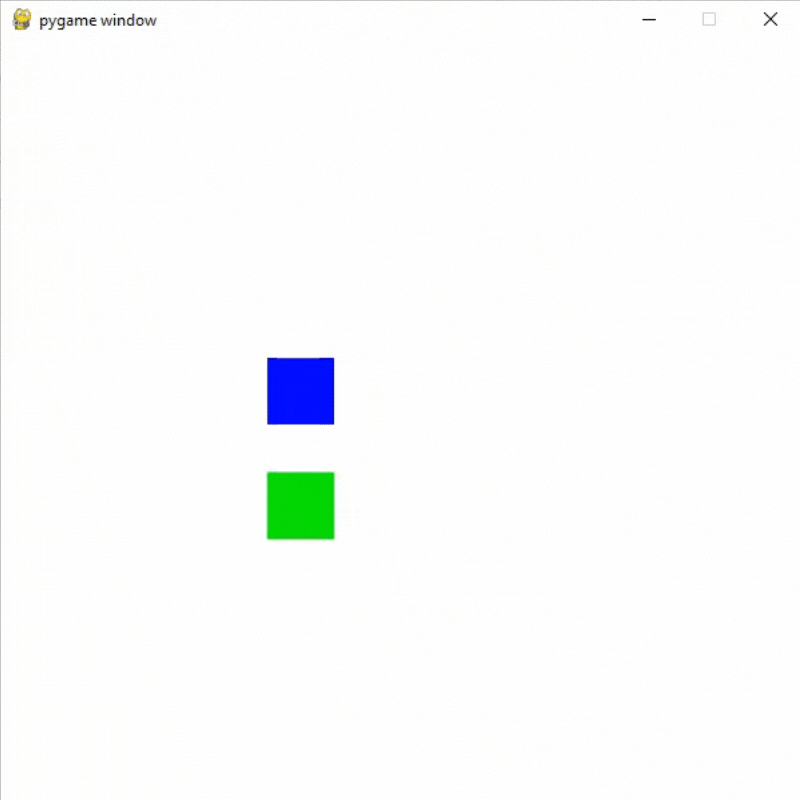Related Articles

# Adding Collisions Using pygame.Rect.colliderect in Pygame

• Difficulty Level : Hard
• Last Updated : 24 Sep, 2021

Prerequisite: Drawing shapes in Pygame, Introduction to pygame

In this article, we are going to use pygame.Rect.colliderect for adding collision in a shape using Pygame in Python.

We can easily add collisions in Pygame shapes using the colliderect( ). For this, we are going to draw two rectangles then we will check if the rectangles are colliding or not.

Syntax: pygame.Rect.colliderect(rect1, rect2)

Parameters: It will take two rects as its parameters.

Returns: Returns true if any portion of either rectangle overlap.

## Python3

 `# Importing the pygame module``import` `pygame``from` `pygame.``locals` `import` `*` `# Initiate pygame and give permission``# to use pygame's functionality``pygame.init()` `# Create a display surface object``# of specific dimension``window ``=` `pygame.display.set_mode((``600``, ``600``))` `# Creating a new clock object to``# track the amount of time``clock ``=` `pygame.time.Clock()`  `# Creating a new rect for first object``player_rect ``=` `Rect(``200``, ``500``, ``50``, ``50``)` `# Creating a new rect for second object``player_rect2 ``=` `Rect(``200``, ``0``, ``50``, ``50``)` `# Creating variable for gravity``gravity ``=` `4` `# Creating a boolean variable that``# we will use to run the while loop``run ``=` `True` `# Creating an infinite loop``# to run our game``while` `run:` `    ``# Setting the framerate to 60fps``    ``clock.tick(``60``)` `    ``# Adding gravity in player_rect2``    ``player_rect2.bottom ``+``=` `gravity` `    ``# Checking if player is colliding``    ``# with platform or not using the``    ``# colliderect() method.``    ``# It will return a boolean value``    ``collide ``=` `pygame.Rect.colliderect(player_rect, p``                                      ``layer_rect2)` `    ``# If the objects are colliding``    ``# then changing the y coordinate``    ``if` `collide:``        ``player_rect2.bottom ``=` `player_rect.top` `    ``# Drawing player rect``    ``pygame.draw.rect(window, (``0``,   ``255``,   ``0``),``                     ``player_rect)``    ``# Drawing player rect2``    ``pygame.draw.rect(window, (``0``,   ``0``,   ``255``),``                     ``player_rect2)` `    ``# Updating the display surface``    ``pygame.display.update()` `    ``# Filling the window with white color``    ``window.fill((``255``, ``255``, ``255``))`

Output:## Python3

 `# Importing the pygame module``import` `pygame``from` `pygame.``locals` `import` `*` `# Initiate pygame and give permission``# to use pygame's functionality``pygame.init()` `# Create a display surface object``# of specific dimension``window ``=` `pygame.display.set_mode((``600``, ``600``))` `# Creating a new clock object to``# track the amount of time``clock ``=` `pygame.time.Clock()`  `# Creating a new rect for first object``player_rect ``=` `Rect(``200``, ``500``, ``50``, ``50``)` `# Creating a new rect for second object``player_rect2 ``=` `Rect(``200``, ``0``, ``50``, ``50``)`  `# Creating a boolean variable that``# we will use to run the while loop``run ``=` `True` `# Speed for the objects``speed_a ``=` `8``speed_b ``=` `-``7` `# Creating an infinite loop``# to run our game``while` `run:` `    ``# Setting the framerate to 60fps``    ``clock.tick(``60``)` `    ``# Adding speed in player rects``    ``player_rect.bottom ``+``=` `speed_a``    ``player_rect2.top ``+``=` `speed_b` `    ``# Checking if player is colliding``    ``# with platform or not using the``    ``# colliderect() method.``    ``# It will return a boolean value``    ``collide ``=` `pygame.Rect.colliderect(player_rect,``                                      ``player_rect2)` `    ``# If the objects are colliding``    ``# then changing the speed direction``    ``if` `collide:``        ``speed_a ``*``=` `-``1``        ``speed_b ``*``=` `-``1` `    ``# Changing the direction if the objects``    ``# goes outside the window``    ``if` `player_rect.top<``0` `or` `player_rect.bottom > ``600``:``        ``speed_a ``*``=` `-``1``    ``if` `player_rect2.bottom > ``600` `or` `player_rect2.top<``0``:``        ``speed_b ``*``=` `-``1``    ` `    ``# Drawing player rect``    ``pygame.draw.rect(window, (``0``,   ``255``,   ``0``),``                     ``player_rect)``    ``# Drawing player rect2``    ``pygame.draw.rect(window, (``0``,   ``0``,   ``255``),``                     ``player_rect2)` `    ``# Updating the display surface``    ``pygame.display.update()` `    ``# Filling the window with white color``    ``window.fill((``255``, ``255``, ``255``))`

Output:Attention geek! Strengthen your foundations with the Python Programming Foundation Course and learn the basics.

To begin with, your interview preparations Enhance your Data Structures concepts with the Python DS Course. And to begin with your Machine Learning Journey, join the Machine Learning – Basic Level Course

My Personal Notes arrow_drop_up